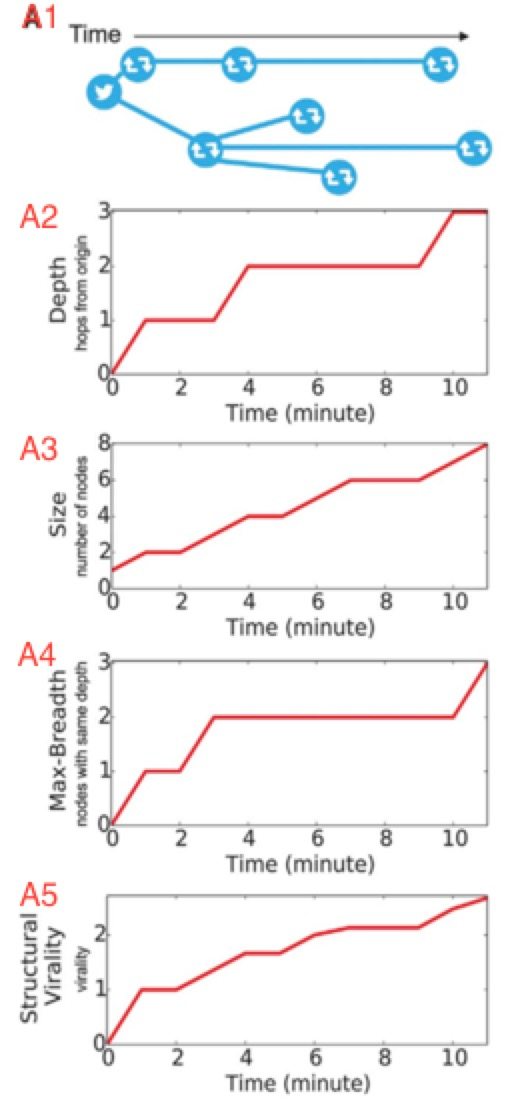3个回答Python绘制折线图、散点图
Python绘图需要下载安装matplotlib模块，它是一个数学绘图库，我们将使用它来制作简单的图表，如折线图和散点图。关于matplotlib模块的下载安装预配置将在后面具体介绍。 （一）绘制折线图 首先，我们先绘制一个简单的折线图 # 调用模块 import matplotlib.pyplot as plt squares=[1, 4, 9, 16, 25] x=[1,...
python绘制折线图示例
python绘制折线图示例
python之折线图绘制体验

python 绘制简易折线图
# 简易折线图绘制 # encoding=utf-8 # 引入对应库文件 import numpy as np import matplotlib.pyplot as plt x = np.linspace(0, 20 ,100) # 类似于平滑度 0表示x坐标原点的值,20表示x的最大值,100表示将值分成100份 y = x + 2 #设置x,y关系 plt.plot(x, y , 'b...
python绘制折线图（多条）
# encoding=utf-8 from matplotlib import pyplot import matplotlib.pyplot as plt names = range(8,21) names = [str(x) for x in list(names)] x = range(len(names)) y_train = [0.840,0.839,0.834,0.832,0.8...
Python绘制简单的折线图
import csv import math import numpy as np # 导入模板pyplot并指定别名plt,pyplot包含很多生成图表的函数 import matplotlib.pyplot as plt # 创建2个列表 input_values = [2, 4, 6, 8, 10] squares = [4, 16, 36, 9, 25] # 传输入值x和输出y值给s...

python使用matplotlib绘制折线图教程
python使用matplotlib绘制折线图教程 Matplotlib是一个Python工具箱，用于科学计算的数据可视化。借助它，Python可以绘制如Matlab和Octave多种多样的数据图形。下面这篇文章主要介绍了python使用matplotlib如何绘制折线图的方法教程，需要的朋友可以参考借鉴。   matplotlib简介 matplo...
QCustomPlot 使用——绘制折线图
//初始化数据 QVector<double> temp; for (int var = 0; var < 25; ++var) { if (rand()%2) { temp.append(var); }else{ temp.append(20); } } QVector<

// 要绘制的四条折线的名字 String[] titles = new String[] { "Crete", "Corfu", "Thassos", "Skiathos" }; // 折线图的x坐标 List<double[]> x = new ArrayList<double[]>(); for (

15 使用Python绘制简单的折线图
2019年2月份编程语言排行榜出炉,多年王者java正式跌落下神坛,Python等上NO.1,Python语言相对java语言它简单,入门更快 对java熟悉的人,上手Python就比较容易了,Python中变量命名,继承等相比java更简单;通过一两个月的学习,发现它太简便了,同样的一个功能,java实现得几十甚至上百行代码实现,而Python只需一行代码搞定,提升了开发效率. ...

MATLAB2017a如何绘制折线图

Python matplotlib绘制折线图简单实现
Python matplotlib绘制折线图简单实现

#encoding:utf-8#使用matplotlib绘制简单的折线图 import matplotlib.pyplot as plt#绘制简单的折线图 squares = [1, 4, 9, 16, 25] plt.plot(squares) #plot函数根据数字绘制出有意义的图形 plt.show() #show函数打开matplotlib查看器,并显示绘制的图形#修改

ChartDirector如何绘制多张折线图？

Python绘制折线图、直方图、散点图、盒图
#第1题折现图 import pandas as pd import numpy as np import matplotlib.pyplot as plt Money=pd.read_csv('Money.csv',sep=',',header=0,index_col='date') axis1=plt.subplot() axis1.plot(Money.index,Money.m...
Python数据可视化库-Matplotlib——折线图绘制
# coding:utf-8 import pandas as pd import numpy as np from matplotlib import pylab as plt # 导入数据可视化库Matplotlib并重命名为plt   plt.rcParams['font.sans-serif']=['SimHei'] # 用来正常显示中文标签 plt.rcParams['axe...
Python数据可视化学习笔记1-绘制折线图
import matplotlib.pyplot as plt input_values = [1,2,3,4,5] squares = [1,4,9,16,25] plt.plot(input_values,squares,linewidth = 5) plt.title('Square Numbers',fontsize =24) plt.xlabel('Value',fontsize ...
Python 时间数据处理和绘制柱状图、散点图、折线图
23/100 “qq_30008595” Python 数据处理和绘制柱状图、散点图 引入库 引入库的列表 import xlrd,os import time import datetime import numpy as np from xlrd import xldate_as_tuple import collections import matplotlib.pyplot as plt ...

python matplotlib从文件中读取数据绘制折线图

Python学习之matplotlib 绘制折线图详解

# 解析tid数据并绘制折线图 # encoding=utf-8 # 引入对应库文件 import numpy as np import matplotlib.pyplot as plt #读取txt文件 f = open('C:/Users/Administrator/Desktop/PythonTool/单波束数据/20180529-0605.tid','r') list = [] #设置...
31、python绘制折线图方法、案例

Python matplotlib，数据可视化，绘制折线图，plot

python数据可视化——利用pyplot绘制折线图和散点图
1、绘制简单折线 #a.py import matplotlib.pyplot as plt squares=[1,4,9,16,25] plt.plot(squares) plt.show() #函数plot()尝试根据数字绘制出有意义的图形 #plt.show()打开matplotlib查看器，并显示绘制的图形 2、修改标签文字和线条粗细 1）使用参数linewidth决

Python使用matplotlib模块绘制多条折线图、散点图

MSchart绘制折线图
MSchart控件折线图表加虚线以及数据点换颜色，有需要的朋友可以下载看看，欢迎评价，还请各位多多指教